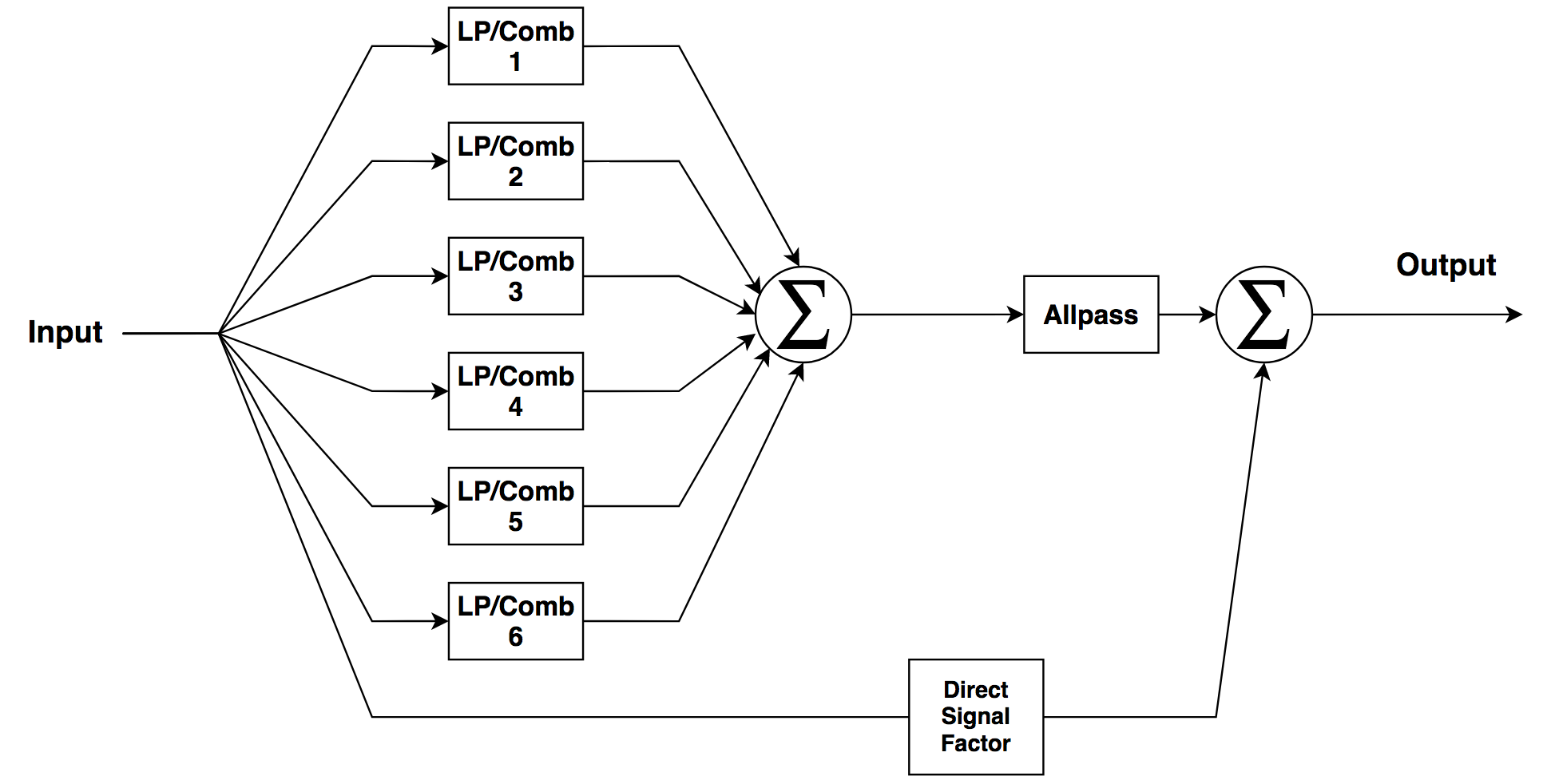# Reverb Demo

### Moorer Reverb App

This application is a demo of a reverb filter structure proposed by J.A. Moorer as detailed by Ken Steiglitz in his book A Digital Signal Processing Primer.

As seen in the diagram below, it utilizes six combination low-pass / comb filters which then feed into an allpass filter. The output of that filter is then mixed back with some of the direct signal.(Click image to Enlarge)

The filters are defined as follows:

Low-pass / comb:
$$\mathcal{H}(z)=\frac{z^{-L}}{1\,-\,R\Big[\frac{1}{1-gz^{-1}}\Big]z^{-L}}$$

Allpass:
$$\mathcal{H}(z)=\frac{z^{-m}\,+\,a}{1\,+\, az^{-m}}$$

While this demo uses the delays and gain coefficients proposed by Moorer as defaults, the application allows the user to modify these values. The user can then listen to the effects of their changes, as well as view the processed waveform and the impulse response of the reverb.